The theoretically preferable approach to recording amortization is the effective-interest method. Interest expense is a constant percentage of the bond’s carrying value, rather than an equal dollar amount each year. The theoretical merit rests on the fact that the interest calculation aligns with the basis on which the bond was priced.

Interest expense is calculated as the effective-interest rate times the bond’s carrying value for each period. The amount of amortization is the difference between the cash paid for interest and the calculated amount of bond interest expense.

Recall that when Schultz issued its bonds to yield 6%, it received \$108,530. Thus, effective interest for the first six months is \$108,530 X 6% X 6/12 = \$3,255.90. Of this amount, \$4,000 is paid in cash and \$744.10 (\$4,000 – \$3,255.90) is premium amortization. The premium amortization reduces the net book value of the debt to \$107,785.90 (\$108,530 – \$744.10). This new balance would then be used to calculate the effective interest for the next period. This process would be repeated each period, as shown in the following table: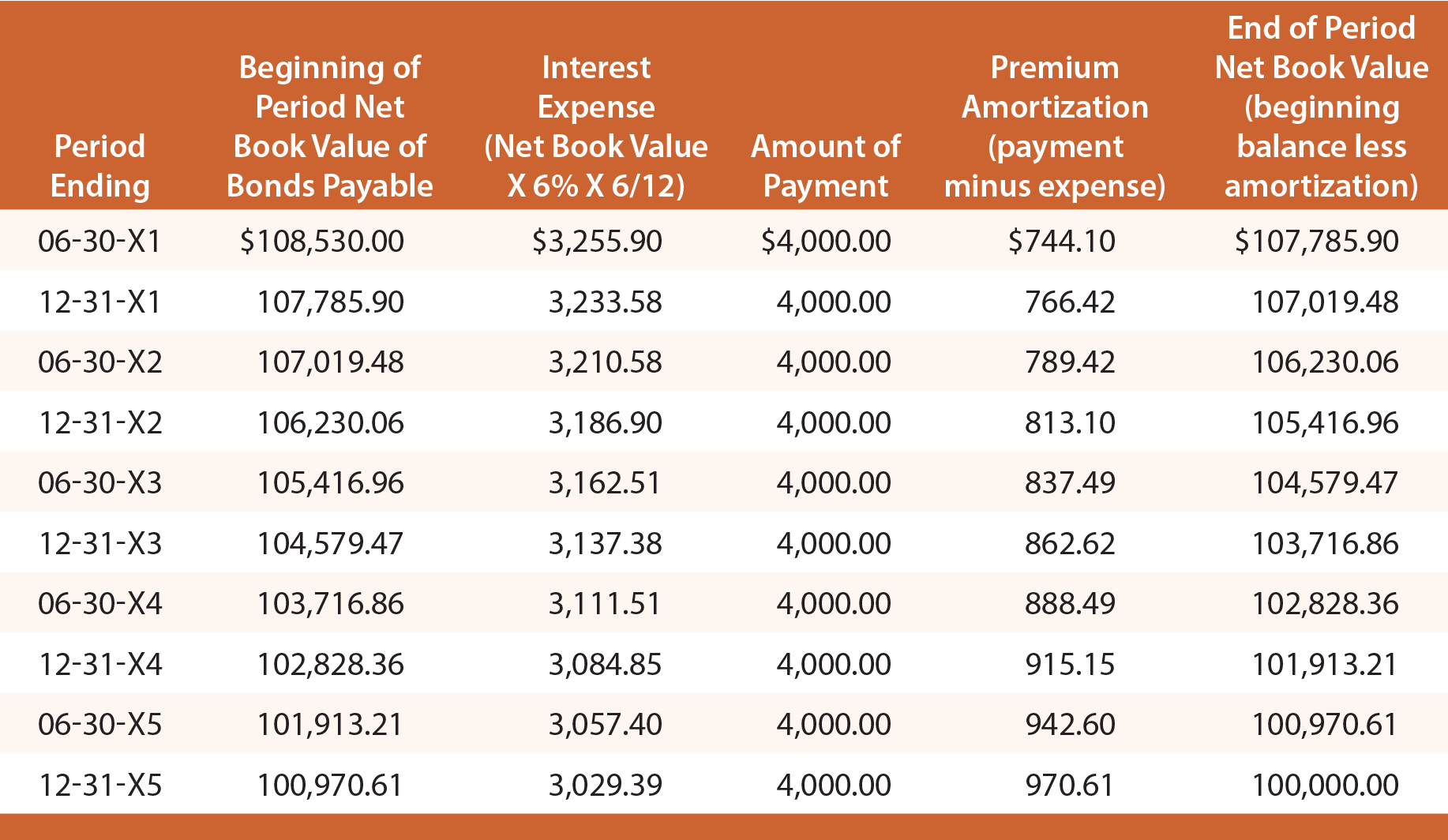The initial journal entry to record the issuance of the bonds, and the final journal entry to record repayment at maturity would be identical to those demonstrated for the straight-line method. However, each journal entry to record the periodic interest expense recognition would vary and can be determined by reference to the preceding amortization table.

The following entry would record interest on June 30, 20X3: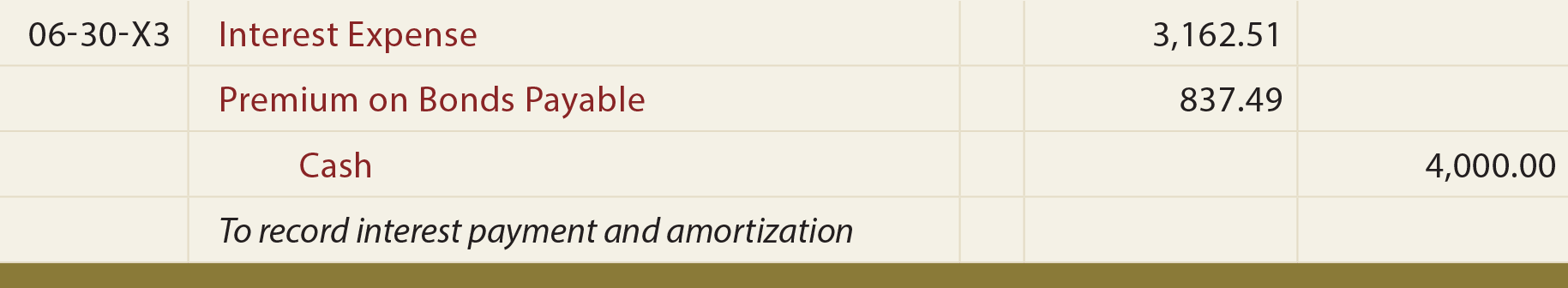The following balance sheet disclosure would be appropriate as of June 30, 20X3: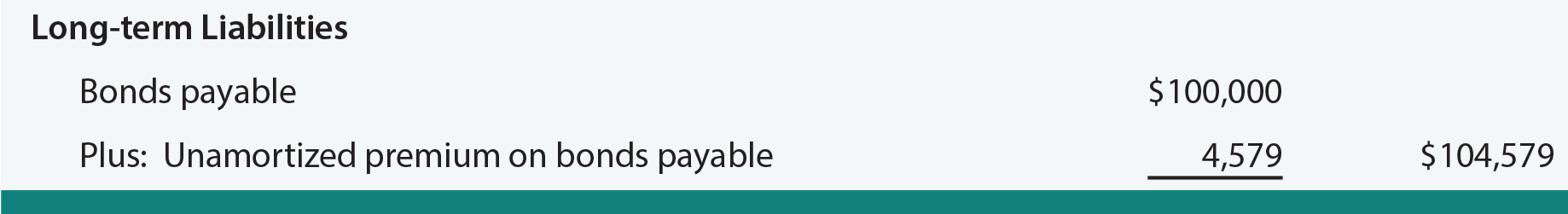### Discount Example

Recall that when Schultz issued its bonds to yield 10%, it received only \$92,278. Thus, effective interest for the first six months is \$92,278 X 10% X 6/12 = \$4,613.90. Of this amount, \$4,000 is paid in cash, and \$613.90 is discount amortization. The discount amortization increases the net book value of the debt to \$92,891.90 (\$92,278.00 + \$613.90). This new balance would then be used to calculate the effective interest for the next period. This process would repeat each period as shown:Each journal entry to record the periodic interest expense recognition would vary, and can be determined by reference to the preceding amortization table. For instance, the following entry would record interest on June 30, 20X3, and result in the balance sheet disclosure below: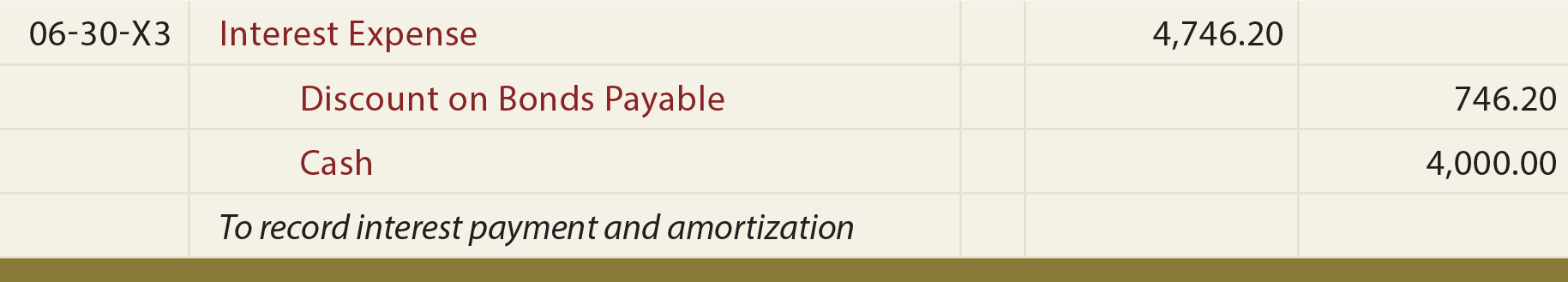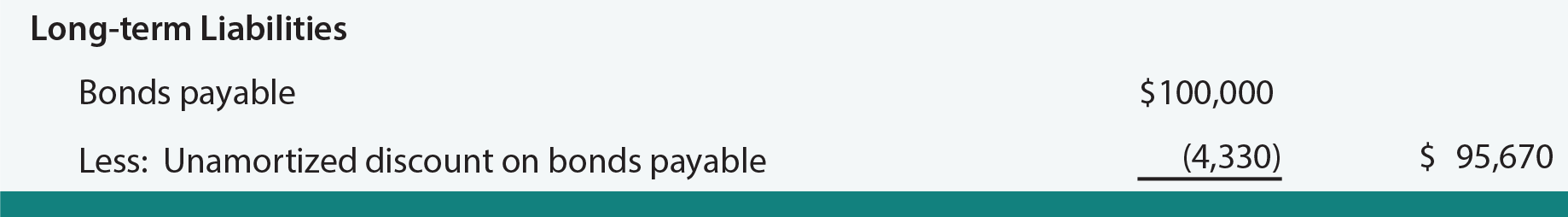Did you learn?
Use the effective-interest method to account for a bond issued at a premium.
Use the effective-interest method to account for a bond issued at a discount.